# Steenrod-Sitnikov homology

(diff) ← Older revision | Latest revision (diff) | Newer revision → (diff)

A homology theory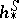supposed to be defined on the category of pairs of compact metric (i.e., metrizable) spaces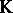, satisfying all Steenrod–Eilenberg axioms (in the case of generalized Steenrod–Sitnikov homology, without a dimension axiom) together with a strong excision axiom (i.e.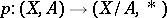induces an isomorphism in homology).

For an ordinary Steenrod–Sitnikov homology theory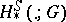(an Abelian group) J. Milnor [a3] established the following axiomatic characterization: An ordinary homology theoryonsatisfying the Eilenberg–Steenrod axioms (with strong excision and dimension axiom) and in addition the cluster (or strong wedge) axiom is isomorphic (as a homology theory) to.

Let,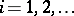, be a family of based spaces in; then the cluster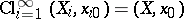is the wedge of the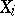, equipped with the strong topology (a neighbourhood of the basepointcontains almost allor, alternatively,). The projection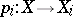induces a mapping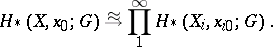(a1)

The cluster axiom requires that (a1) be an isomorphism.

The cluster axiom turns out to be some kind of continuity axiom: A homology theory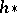is continuous whenever there is a natural isomorphism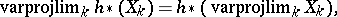(a2)

i.e. the functorcommutes with inverse limits. S. Eilenberg and N. Steenrod [a2] discovered that there is a conflict between (a2) and the exactness axiom, so that no homology theory can be continuous. However, the cluster axiom reveals itself as a special case of (a2): One has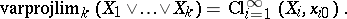and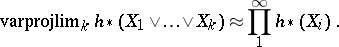Milnor's uniqueness theorem admits an extension for generalized homology theories [a1]: A homology theoryonsatisfying the Milnor axioms (now without a dimension axiom) is, up to an isomorphism of homology theories, uniquely determined by its restriction to the category of compact absolute neighbourhood retracts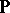(or polyhedra or CW-spaces). Moreover, every homology theoryonadmits a unique extensionoveras a Steenrod–Sitnikov homology theory. If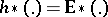(the homology theory with coefficients in a spectrum,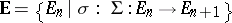) (cf. also Spectrum of spaces), then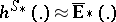is uniquely determined by the spectrum.

It turns out [a1] that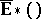is constructed like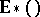, but with replacing continuous mappings by strong shape mappings. So, Steenrod–Sitnikov homology is defined on a strong shape category (cf. Strong shape theory).

Steenrod–Sitnikov homology appears as the appropriate tool for handling geometric problems in. This pertains to Alexander duality in its most modern form, dealing with homology and cohomology with coefficients in a spectrum, to-duality, as well as to dimension theory of subspaces of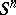.

Ordinary Steenrod–Sitnikov homology appeared for the first time in [a6] as a tool for Alexander duality. In the 1950s, K. Sitnikov rediscovered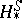by using a different definition [a4], [a5], without knowing about [a6], and verified an Alexander duality theorem for arbitrary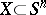by using this kind of homology with compact support.

As official opponent in Sitnikov's doctoral dissertation, G.S. Chogoshvili pointed out that the homology groups of Sitnikov and Steenrod agree.

Sitnikov's definition ofis modelled after the classical definition of Vietoris homology: A Vietoris cycle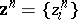on a space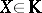consists of a sequence of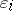-cycles,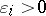(i.e. each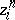is a cycle with simplices with vertices inof maximal diameter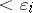), with, such that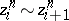by some-chain: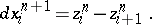A Sitnikov-cycle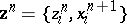specifies the chains. So, two Sitnikov cyclesgive rise to the same Vietoris cycle but eventually to different Sitnikov homology classes.

There have been attempts to extend Steenrod–Sitnikov homology to more general topological spaces, leading to what is called strong homology. This was initiated for ordinary homology theories by S. Mardešić and Ju. Lisica. There is an analogous axiomatic characterization of strong homology, where one has to replace the cluster axiom by a continuity axiom on the chain level (the c-continuity of a homology theory).

How to Cite This Entry:
Steenrod-Sitnikov homology. Encyclopedia of Mathematics. URL: http://encyclopediaofmath.org/index.php?title=Steenrod-Sitnikov_homology&oldid=17110
This article was adapted from an original article by F.W. Bauer (originator), which appeared in Encyclopedia of Mathematics - ISBN 1402006098. See original article